## 3. 1. 2 Re-Formulation on Topological Properties

The indices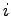and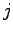from (3.2) and (3.3) refer to the system matrix or to the numbering of the degrees of freedom in the function space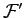. In this derivation, instead of indices, topological entities, in this simple case vertices, are used. Therefore, each function of the function spacecan be directly associated with its corresponding vertex so that one can write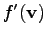instead of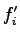, whereis the vertex with index. In analogy, a function of the function space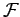can be written as restriction of a function corresponding to a vertexrestricted on a cell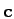, namely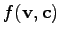. It has to be assured that the vertexand the cellare incident in order to retrieve a valid, non-zero basis function of. As can be seen easily, these considerations can be used analogously for other topological entities on which quantities are stored. The substitution of the notation leads to the following formulation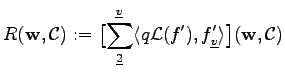(3.4)

Next, the shape functions as well as the weighting functions, both from the function spaceare written as functions of the function space. This can only be applied, if the function space is derived from a tesselation of the underlying simulation domain and yields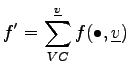(3.5)

with the resudial term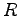and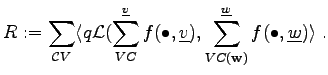(3.6)

As the summands of the inner sums do not depend on the summation variables of the other summation, the sums can be written in the following manner: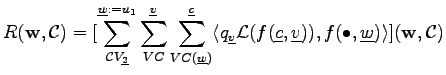(3.7)

Under the assumption that two functions of, namely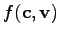and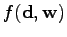can only yield a non-zero inner product,has to be identical to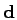. Otherwise at least one of the functions is identically zero on the complete simulation domain. Furthermore, both of the verticesand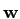have to be incident with the common cell, because of the definition of the function space. Figures 3.1 and 3.2 show the traversal mechanisms that have to be available for the implementation of finite element schemes.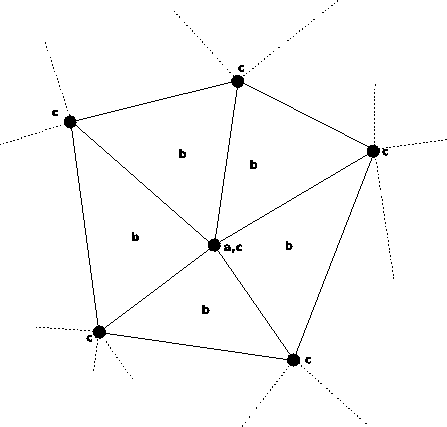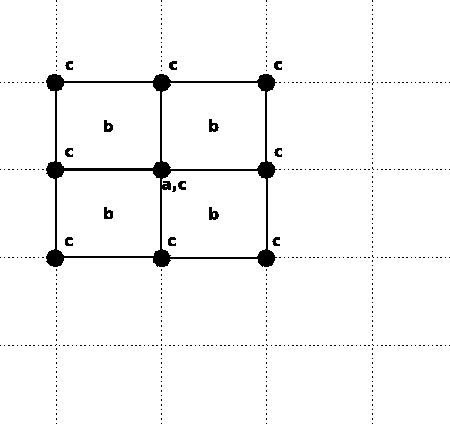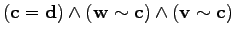(3.8)

Under the assumption of condition (3.8) the summation can be simplified to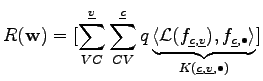(3.9)

The quadrature of the respective shape functions can be performed in a straight forward manner. Moreover, different differential equations, quadrature methods shape functions, weighting functions quadrature methods and even inner products can be chosen without affecting the overall algebraic structure of the equation system. The specialization to a certain set of methods can be reduced to formulating a proper coefficient function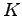.

Michael 2008-01-16